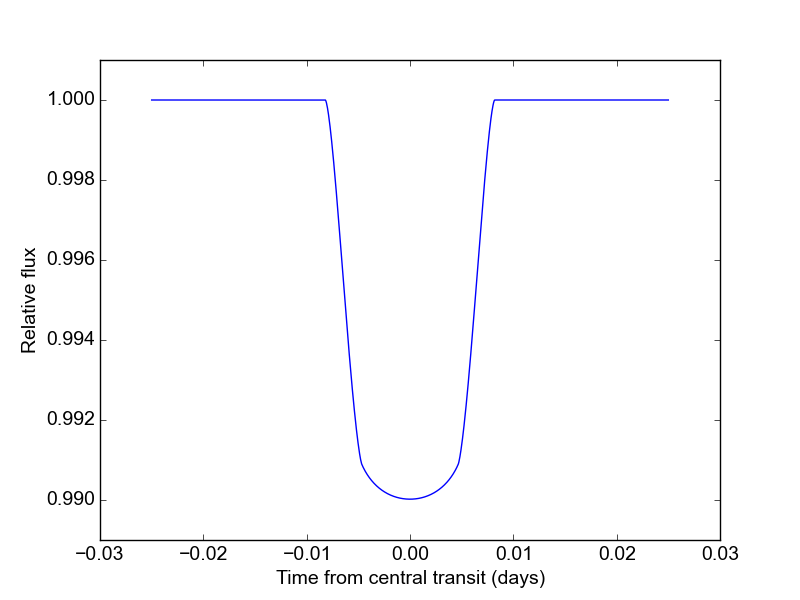# Quickstart¶

If you’re in a hurry to model some awesome transits, you’re on the right page. Here’s an example of basic robin usage to calculate a model light curve with quadratic limb darkening. (For more detailed examples, check out the Tutorial.)

First, we import robin and a few of the usual packages:

import robin
import numpy as np
import matplotlib.pyplot as plt


Next we create a TransitParams object to store the physical parameters describing the transit:

params = robin.TransitParams()
params.t0 = 0.                       #time of inferior conjunction
params.per = 1.                      #orbital period
params.rp = 0.1                      #planet radius (in units of stellar radii)
params.a = 15.                       #semi-major axis (in units of stellar radii)
params.inc = 87.                     #orbital inclination (in degrees)
params.ecc = 0.                      #eccentricity
params.w = 90.                       #longitude of periastron (in degrees)
params.u = [0.1, 0.3]                #limb darkening coefficients [u1, u2]
params.limb_dark = "quadratic"       #limb darkening model


Note that for circular orbits, robin uses the convention params.w = 90. The units for params.t0 and params.per can be anything as long as they are consistent.

We also need to specify the times at which we wish to calculate the model:

t = np.linspace(-0.05, 0.05, 100)


Using these parameters, we initialize the model and calculate a model light curve:

m = robin.TransitModel(params, t)    #initializes model
flux = m.light_curve(params)          #calculates light curve


Voilà! Here’s a figure showing the light curves:

plt.plot(t, flux)
plt.xlabel("Time from central transit")
plt.ylabel("Relative flux")
plt.show()This code is available in full at https://github.com/bmorris3/robin/tree/master/docs/quickstart.py.